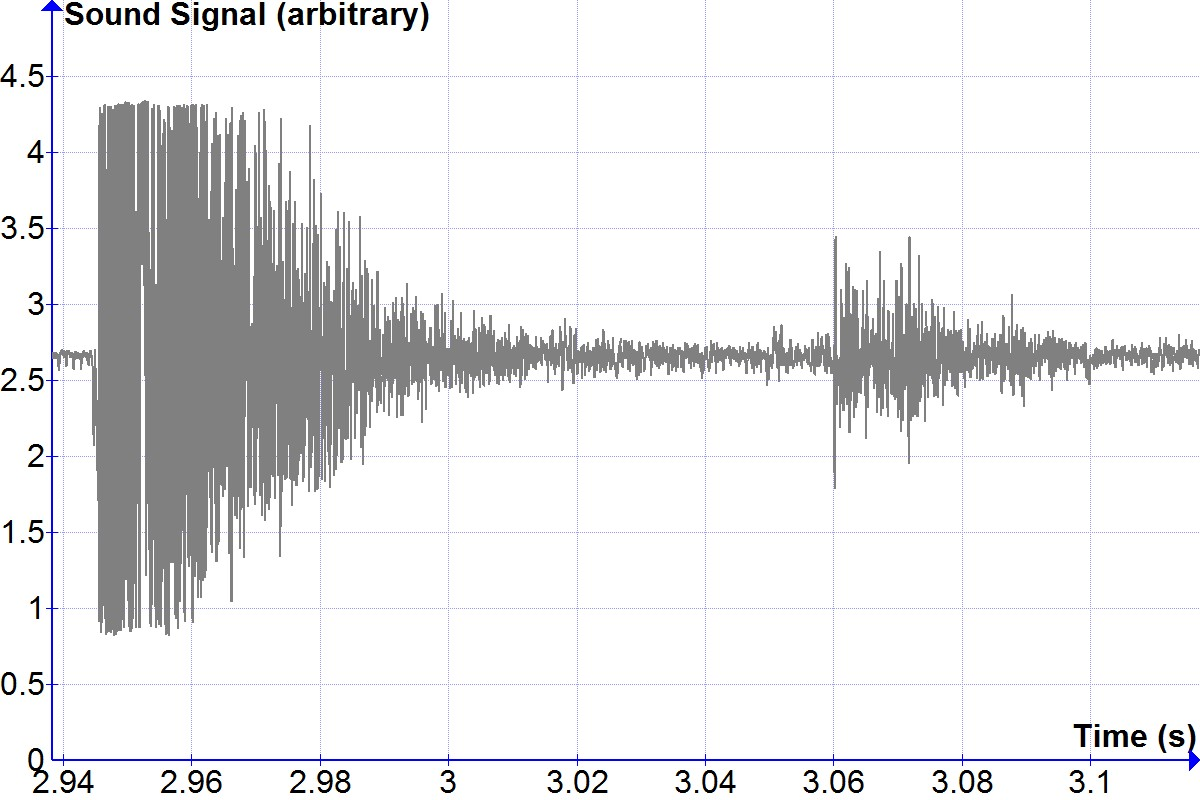# Direct Echo-Based Measurement of the Speed of Sound

[Total: 6    Average: 4/5]Figure 1

Figure 1: Distance vs. time a firecracker report echoed off of a building at different distances on both a cold and a warm day.

The speed of sound varies slightly with temperature, but at constant temperature, the distance sound travels increases linearly with time according to the equation, D = Vt, where D is the distance traveled (in meters), V is the speed of sound (in m/s), and t is the time in seconds. In this experiment, the time for the report of a firecracker to travel specific round trip distances will be measured as a test of the above formula. Hypothesis: The distance sound travels is linear in time, according to D = Vt, and the velocity is well approximated by accounting for temperature.

Data was acquired by setting a fire cracker a series of carefully measured distances from a large flat wall and igniting the firecracker a short distance from a microphone while digitally recording the microphone signal. Here, a Vernier LabPro was used with a microphone sampling at 20000 samples per second, but other sound digitizers can also be used.  In this case, it was convenient to use a nearby church building for the large flat wall, as shown in Figure 2.Figure 2

Figure 2:  Large, flat wall used to provide echos.  Note, it is best to use the side of the building so that the intended wall is the only surface reflecting sound waves back to the microphone.

The initial report and the echo off the wall are easily visible in the data when graphed, as shown in Figure 3. The time between these two signals is the round trip travel time of the sound to the wall and back (keep in mind that the travel distance is TWICE the distance of the firecracker from the wall).Figure 3

Figure 3: Sound signal showing direct firecracker report close to 2.945 s and the reflected report near 3.06 s.

Each file was graphed to determine the direct report time and the echo time. The difference is the round trip travel time.  Then the distance data is plotted vs. the time data in graph.exe. (https://www.padowan.dk/download/)  When fitting to a trendline in graph.exe, we were sure to check the box to set the vertical intercept to zero, as the hypothesis predicts not only a linear relationship, but also a vertical intercept of zero (a direct proportionality.)

Inspection of Figure 1 shows that the hypothesis was supported.  Comparison with the speeds of sound predicted at the two temperatures is in agreement to within about 1%.  (For example: https://www.weather.gov/epz/wxcalc_speedofsound ) It may be noted that a couple other approaches to analysis are possible.  A speed can be computed for each trial as the distance divided by the time for that trial.  Then each of the five resulting speeds can be averaged, and a standard deviation and standard error of the mean (SEM) computed with standard spreadsheet calls.  This approach provides a speed of sound measurement very close to the least squares fit, and also provides an uncertainty estimate (the SEM).  Another analysis approach is to use the LINEST spreadsheet call to do the least squares fit.  An advantage of this method is the LINEST spreadsheet call can also return an estimated uncertainty for the slope in the fit, which is an estimate of the uncertainty in the velocity, since the slope IS the velocity.

This lab was completed in both a early high school Physical Science class and a late high school Physics class last year at a school where I served as a volunteer lab coordinator for several labs.  The students, the teachers, and the administrators at the school all got a bang out of it.  Since it was a favorite, I returned to reprise the lab at the end of the year on a warm day which then provided an opportunity to confirm the expected dependence of the speed of sound on temperature.  Fun times!

Tags:
39 replies